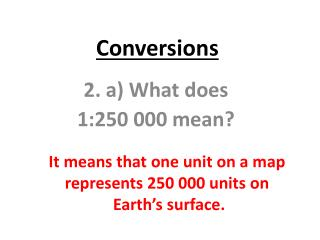DownloadDownload PresentationConversions

# Conversions

Télécharger la présentation## Conversions

- - - - - - - - - - - - - - - - - - - - - - - - - - - E N D - - - - - - - - - - - - - - - - - - - - - - - - - - -
##### Presentation Transcript

1. Conversions 2. a) What does 1:250 000 mean? It means that one unit on a map represents 250 000 units on Earth’s surface.

2. Conversions continued 2. b) What does 1:3 000 000 mean? It means that one unit on a map represents 300 000 units on Earth’s surface.

3. One centimetre on the map represents 0.5 km on Earth’s surface. 3. a) What does 1 cm to 0.5 km mean?

4. 4) The direct statement scale of a map is 1 cm to 35 km. If the map distance between points A and B is 9 cm, what is the actual distance? 1 cm = 35 km (9 x 1) cm = (9 x 35) km 9 cm = 315 km The actual distance between points A and B is 315 km.

5. You try #5 by yourself!

6. 5) The direct statement scale of a map is 1 cm to 250 km. If two places are 17 cm apart on the map, what is the actual distance between them? 1 cm = 250 km (17 x 1) cm = (17 x 250) km 17 cm = 4250 km The actual distance between the two places is 4250 km.

7. Homework: Page 48 #6 (a, b, c) *You will need a ruler in order to measure out the centimeters!